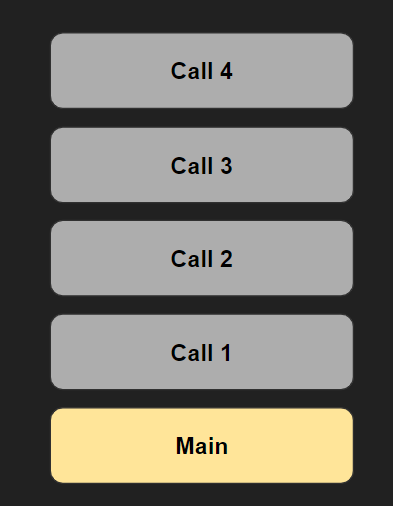Learnearn.uk » A Level Computer Science Home » Recursion

# Recursion

## Intro

### What is recursion?Recursion in Computer Science is where a function calls itself. When a function is is called recursively an extra frame(layer) is added to the stack, with each subsequent frame being added on top. Recursion will continue until the base case is reached, at which point the inner most call will return and the top frame removed from the stack.

In order to be successfully recursive a recursive function must obey 3 rules:

3 Rules of recursion

• The algorithm must have a base case
• The algorithm must work towards the base case
• The algorithm must call itself recursively

Why use recursion?

Recursion is useful in many problems where iteration would prove difficult or impossible.

Stacks and Unwinding

Unwinding is the process of returning from one stack from back down to the next, and so on until recursion is complete.

Compiler and the stack – what does it have to do?

Stack Overflow Exceptions & Recursion Depth  LimitsEvery time a function enters another level of recursion an extra frame is added to the call stack. In order to process the frame  an area of memory has to be allocated. Eventually the memory allocated for recursion is exhausted and the Recursive Depth Limit is reached. If the program attempts a further recursive call then a Stack Overflow Exception Error will be raised.

## Factorial

### Factorial Example

This trivial example uses recursion to calculate the factorial of a number. This is a classic example that often appears on exam paper questions.

The factorial of a number is calculated by multiplying it by all the positive integers smaller than it.

E.g. Factorial of 5 is 5*4*3*2*1 = 120

## Fractal Tree

### Fractal Tree Visual Demonstration

This fractal tree algorithm uses recursion and demonstrates visually how depth-first recursive tree traversal works, as each branch is explored to its fullest depth before moving onto the next branch.

## File Traversal

### File / Folder Tree Traversal

A great example of how tree traversal can be used practically is in file system tree traversal.

The algorithm is supplied with a folder/drive name and the files/folders are explored recursively.

## Binary Search

Binary Search Algorthm

The binary search algorithm can be programmed using a recursive algorithm.

## Brute Force

### Brute Force Password Hacker – For Loop Version

Here is a brute force password hacker that creates a tree of password possibilities. Although this algorithm works as expected it has 2 problems:

1. If the maximum password length is changed from 4 to a larger number you are going to need another nested loop for each extra possible character.
2. It is slightly slower than the recursive version below and would be much slower if a longer password / extra possible characters were used.

### Brute Force Password Hacker – Recursive Version

In this version of the password hacker it uses recursion. As you can see from the code it is both easy to read and to maintain. Changing the maximum password length is a matter of simply changing the assigned value.

## Challenges

### Programming Challenges

Challenge 1 – Sum of List of numbers

Write a program that adds up all the numbers in a list

Challenge 2 – Fibonacci Sequence

Write a program that solves the Fibonacci sequence for a given number.

Challenge 3 – Meal Options

Customers can choose from the follow options each day :

• Pizza
• Spaghetti
• Curry
• Hamburger

Use recursion to generate a list of all the possible combinations that a customer could choose over the three days.

Challenge 4 – File Search

Adapt the File Tree traversal program so that you can use it to search for a file name and it will print out the location of all files with that name.

Challenge 5 – Adapt the tree traversal programA colourful implementation of the recursive tree algorithm

• Adapt the program so that the leaves of the branch are green

Further Challenges and Ideas

More Maths based Recursion Programs

List of other common recursion problems

## Resources

### Resources

Teacher Slideshow Document

What is recursion ComputerPhile Video

Tail Call Optimization / Stack Overflow Song (Extension Task)

Online Python Visualiser

Isaac Computer Science Page

Theory Revision Activity – Recursion Requirements

Work through each of the 6  examples of recursion (Factorial, Fractal Tree, File Traversal, Binary Search, Brute Force) and for each example put together a page on a document detailing how the example satisfies the rules of recursion. See this example.

Past Paper Questions

W18 Paper 41 – Qn 4